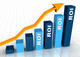# Return on Investment

By Research DeskReturn on Investment (RoI) measures rupee earned from each rupee invested for a particular time period. A higher positive RoI indicates investment gains compared to investment costs. Thus, investors should invest their money in the highest positive RoI. RoI is expressed as a percentage.

ROI = Net Profit / Total Investment * 100

Where,

Net Profit = Profit after tax (PAT)

The formula can also be modified to Gain from investment less cost of investment divided by cost of investment.

ROI = (Gains from Investment - Cost of Investment) / Cost of Investment

Let us take an example of where an investor purchased 50 shares of Page Industries on 1st August 2017 for Rs. 17,000 and sold them on 1st August 2018 for Rs. 30,000.

In this case, the investment made by the investor was Rs. 8,50,000 and the amount that he received when he sold the shares was Rs. 15,00,000.

Return on Investment = (15,00,000 – 8,50,000) / 8,50,000

= 76.47%

Therefore, ROI on Page Industries for this investor was 76.47%.